[image]

# Energy, heat, and temperature

## ... an introduction: how do they differ?"1  What is Energy?Energy is one of the most fundamental and universal concepts of physical science, but one that is remarkably difficult to define in way that is meaningful to most people. This perhaps reflects the fact that energy is not a “thing” that exists by itself, but is rather an attribute of matter (and also of electromagnetic radiation) that can manifest itself in various ways. It can be observed and measured only indirectly through its effects on matter that acquires, loses, or possesses it. [image]

You will recall from earlier science courses that energy can take many forms: mechanical, chemical, electrical, radiation (light), and thermal. You also know that energy is conserved; it can be passed from one object or place to another, but it can never simply disappear.

### Kinetic energy and potential energy

In the 17th Century, the great mathematician Gottfried Leibniz (1646-1716) suggested the distinction between vis viva ("live energy") and vis mortua ("dead energy"), which later became known as kinetic energy and potential energy.

Except for radiant energy that is transmitted through an electromagnetic field, most practical forms of energy we encounter are of two kinds: kinetic and potential.Kinetic energy is associated with the motion of an object; a body with a mass m and moving at a velocity v  possesses the kinetic energy mv2/2.

Worth knowing:  This "v-squared" part is important; if you double your speed, you consume four times as much fuel (glucose for the runner, gasoline or electricity for your car.

Potential energy is energy a body has by virtue of its location in a force field— a gravitational, electrical, or magnetic field. For example, if an object of mass m is raised off the floor to a height h, its potential energy increases by mgh, where g is a proportionality constant known as the acceleration of gravity. Similarly, the potential energy of a particle having an electric charge depends depends on its location in the field, and on the magnitude of the field and the charge.

#### Kinetic and potential energy are freely interconvertible

Pick up a book and hold it above the table top; you have just increased its potential energy in the force field of the earth's gravity.  Now let it drop. Its newly-acquired potential energy begins to re-appear as kinetic energy as it accelerates downward at a velocity increasing by 9.8 m/sec every second
(9.8 m sec–2 or 32 ft sec–2).  At the instant it strikes the surface, the potential energy you gave supplied to the book has now been entirely converted into kinetic energy.

And what happens to that kinetic energy after the book stops moving? It is still there, but you can no longer see its effect; it has now become dispersed as thermal kinetic energy ("heat") into the molecules of the book, the table top, and, ultimately, into the surroundings, including the air.

The more you think about it, the more examples of kinetic-potential conversion you will find in everyday life:

In many other instances, however, the energy of an object can be seen to repeatedly alternate between potential and kinetic forms. Left alone, the process continues indefinitely until friction has dissipated the energy into the surroundings:

### Energy's graveyard: Thermal energy

Energy is conserved: it can neither be created nor destroyed.  But it can, and eventually always will, disappear from our view and into the microscopic world of individual molecular particles.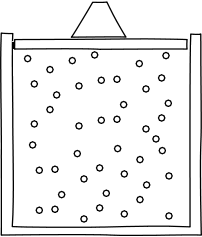All molecules at temperatures above absolute zero are in a continual state of motion, and they therefore possess kinetic energy. But unlike the motion of a massive body such as a baseball or a car that is moving along a defined trajectory, the motions of individual atoms or molecules are random and chaotic, forever changing in magnitude and direction as they collide with each other or (in the case of a gas,) with the walls of the container. [image]The sum total of all of this microscopic-scale randomized kinetic energy within a body is given a special name, thermal energy.

Although we cannot directly see thermal energy in action, we can certainly feel it; as we will see farther on, it correlates directly with the temperature of an object.

### The chemistry connection

Atoms and molecules are the principal actors-out of thermal energy, but they possess other kinds of energy as well that play a major role in chemistry.

#### Bond energy

H2+ is energetically stable enough to exist as an identifiable entity, and thus fits the definition a molecule.  But it is also extremely reactive, so it doesn't sit around for very long.  It can only be observed when a high-voltage electrical discharge is passed through hydrogen gas; the blue glow one sees represents its demise as it picks up electrons and reverts to the far more stable dihydrogen molecule H2.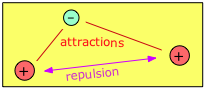Consider, for example, the simplest possible molecule. This is the hydrogen molecule ion, H2+, in which a single electron simultaneously attracts two protons.   These protons, having identical charges, repel each other, but this is overcome by the electron-proton attractions, leading to a net decrease in potential energy when an electron combines with two protons. This potential energy decrease is sufficient to enable H2+ to exist as a discrete molecule which we can represent as [H—H]+ in order to explicitly depict the chemical bond that joins the two atoms.

The strength of a chemical bond increases as the potential energy associated with its formation becomes more negative.

Chemical bonds also possess some kinetic energy that is associated with the "motion" of the electron as it spreads itself into the extended space it occupies in what we call the "bond". This is a quantum effect that has no classical counterpart.  The kinetic energy has only half the magnitude of the potential energy and works against it; the total bond energy is their sum.

#### Chemical energy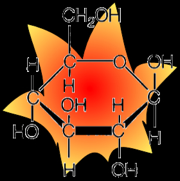The chemical bonds in the glucose molecules store the energy that fuels our bodies.

Molecules are vehicles both for storing and transporting energy, and the means of converting it from one form to another when the formation, breaking, or rearrangement of the chemical bonds within them is accompanied by the uptake or release of energy, most commonly in the form of heat.

Chemical energy refers to the potential and kinetic energy associated with the chemical bonds in a molecule.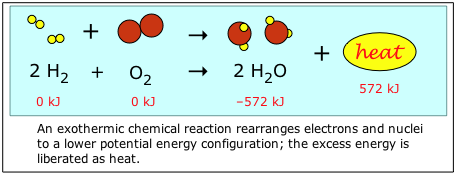Consider what happens when hydrogen and oxygen combine to form water. The reactants H2 and O2 contain more energy in its chemical bonds than does H2O, so when they combine, the excess energy is liberated, given off in the form of thermal energy, or "heat".

By convention, the energy content of the chemical elements in their natural state (H2 and O2 in this example) are defined as "zero". This makes calculations much easier, and gives most compounds negative "energies of formation". (see below)

### Energy scales and units

#### Energy scales are always arbitrary

You might at first think that a book sitting on the table has zero kinetic energy since it is not moving. In truth, however, the earth itself is moving; it is spinning on its axis, it is orbiting the sun, and the sun itself is moving away from the other stars in the general expansion of the universe. Since these motions are normally of no interest to us, we are free to adopt an arbitrary scale in which the velocity of the book is measured with respect to the table; on this so-called laboratory coordinate system, the kinetic energy of the book can be considered zero.We do the same thing with potential energy. If we define the height of the table top as the zero of potential energy, then an object having a mass m suspended at a height h above the table top will have a potential energy of mgh. Now let the object fall; as it accelerates in the earth's gravitational field, its potential energy changes into kinetic energy. An instant before it strikes the table top, this transformation is complete and the kinetic energy ½ mv2 is identical with the original mgh. As the object comes to rest, its kinetic energy appears as heat (in both the object itself and in the table top) as the kinetic energy becomes randomized as thermal energy.

### Energy units

Energy is measured in terms of its ability to perform work or to transfer heat.

Mechanical work is done when a force f displaces an object by a distance d:
w = f × d. The basic unit of energy is the joule. One joule is the amount of work done when a force of 1 newton acts over a distance of 1 m;
thus 1 J = 1 N-m. The newton is the amount of force required to accelerate a 1-kg mass by 1 meter per second in one second: 1 m sec–2, so the basic dimensions of the joule are kg m2 s–2. The other two units in wide use. the calorie and the BTU (British thermal unit) are defined in terms of the heating effect on water. For the moment, we will confine our attention to the joule and calorie.

2  Heat and work

Heat and work are both measured in energy units, but they do not constitute energy itself. As we will explain below, they refer to processes by which energy is transferred to or from something— a block of metal, a motor, or a cup of water.

### What is heat?When a warmer body is brought into contact with a cooler body, thermal energy flows from the warmer one to the cooler until their two temperatures are identical. The warmer body loses a quantity of thermal energy ΔE, and the cooler body acquires the same amount of energy. We describe this process by saying that "ΔE joules of heat has passed from the warmer body to the cooler one." It is important, however, to understand that

Heat is the transfer of energy due to a difference in temperature

We often refer to a "flow" of heat, recalling the 18th-century notion that heat was an actual substance called “caloric” that could flow like a liquid.

In other words, heat is a process; it is not something that can be contained or stored in a body. It is important that you understand this, because the use of the term in our ordinary conversation ("the heat is terrible today") tends to make us forget this distinction.

There are basically three mechanisms by which heat can be transferred: conduction, radiation, and convection.  The latter process occurs when the two different temperatures cause different parts of a fluid to have different densities.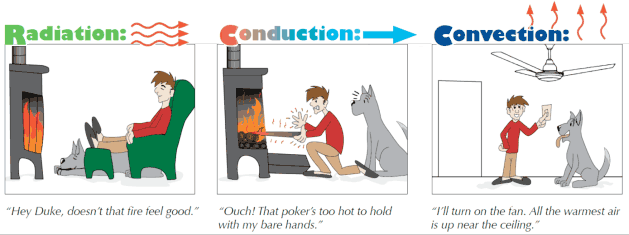[image]

### What is work?Work is the transfer of energy by any process other than heat.

Work, like energy, can take various forms: mechanical, electrical, gravitational, etc. All have in common the fact that they are the product of two factors, an intensity term and a capacity term. For example, the simplest form of mechanical work arises when an object moves a certain distance against an opposing force. Electrical work is done when a body having a certain charge moves through a potential difference.

type of work intensity factor capacity factor formula
mechanical force change in distance f Δx
gravitational gravitational potential (a function of height) mass mgh
electrical potential difference quantity of charge QΔV

Performance of work involves a transformation of energy; thus when a book drops to the floor, gravitational work is done (a mass moves through a gravitational potential difference), and the potential energy the book had before it was dropped is converted into kinetic energy which is ultimately dispersed as thermal energy.Mechanical work is the product of the force exerted on a body and the distance it is moved: 1 N-m = 1 J.

[image]

Heat and work are best thought of as processes by which energy is exchanged, rather than as energy itself. That is, heat “exists” only when it is flowing, work “exists” only when it is being done.

When two bodies are placed in thermal contact and energy flows from the warmer body to the cooler one,we call the process “heat”. A transfer of energy to or from a system by any means other than heat is called “work”.

So you can think of heat and work as just different ways of accomplishing the same thing: the transfer of energy from one place or object to another.

 To make sure you understand this, suppose you are given two identical containers of water at 25°C. Into one container you place an electrical immersion heater until the water has absorbed 100 joules of heat. The second container you stir vigorously until 100 J of work has been performed on it. At the end, both samples of water will have been warmed to the same temperature and will contain the same increased quantity of thermal energy. There is no way you can tell which contains "more work" or "more heat".

#### An important limitation on energy conversion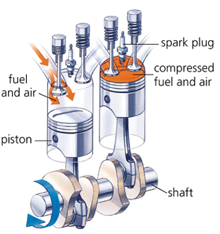An internal combustion engine converts the chemical energy stored in the chemical bonds of its fuel into thermal energy. But only a part of this energy is available to perform work; the remainder is dispersed into the surroundings through the exhaust.

This limitation is the essence of the Second Law of Thermodynamics which we will get to much later in this course.

Thermal energy is very special in one crucial way. All other forms of energy are interconvertible: mechanical energy can be completely converted to electrical energy, and the latter can be completely converted to thermal, as in the water-heating example described above. But although work can be completely converted into thermal energy, complete conversion of thermal energy into work is impossible. A device that partially accomplishes this conversion is known as a heat engine; a steam engine, a jet engine, and the internal combustion engine in a car are well-known examples.

3  Temperature and its meaning

We all have a general idea of what temperature means, and we commonly associate it with "heat", which, as we noted above, is a widely mis-understood word.

Both relate to what we described above as thermal energy—the randomized kinetic energy associated with the various motions of matter at the atomic and molecular levels.

Heat, you will recall, is not something that is "contained within" a body, but is rather a process in which [thermal] energy enters or leaves a body as the result of a temperature difference.

So when you warm up your cup of tea by allowing it to absorb 1000 J of heat from the stove, you can say that the water has acquired 1000 J of energy — but not of heat.  If, instead, you "heat" your tea in a microwave oven, the water acquires its added energy by direct absorption of electromagnetic energy; because this process is not driven by a temperature difference, heat was not involved at al!!

### Thermometry

We commonly measure temperature by means of a thermometer — a device that employs some material possessing a property that varies in direct proportion to the temperature. The most common of these properties are the density of a liquid, the thermal expansion of a metal, or the electrical resistance of a material.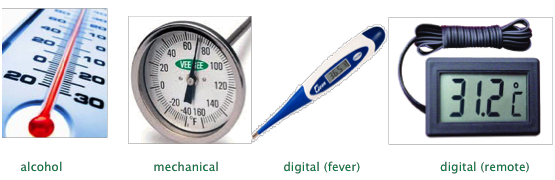The ordinary thermometer we usually think of employs a reservoir of liquid whose thermal expansion (decrease in density) causes it to rise in a capillary tube. Metallic mercury has traditionally been used for this purpose, as has an alcohol (usually isopropyl) containing a red dye.

Mercury was the standard thermometric liquid of choice for more than 200 years, but its use for this purpose has been gradually phased out owing to its neurotoxicity. Although coal-burning, disposal of fluorescent lamps, incineration and battery disposal are major sources of mercury input to the environment, broken thermometers have long been known to release hundreds of tons of mercury. Once spilled, tiny drops of the liquid metal tend to lodge in floor depressions and cracks where they can emit vapor for years.

The Sort of Sad Death of the Mercury Thermometer (Slate Magazine)

### What is temperature?

Temperature is a measure of the average kinetic energy of the molecules within the water. You can think of temperature as an expression of the "intensity" with which the thermal energy in a body manifests itself in terms of chaotic, microscopic molecular motion.

Heat is the quantity of thermal energy that enters or leaves a body.

Temperature measures the average translational kinetic energy of the molecules in a body.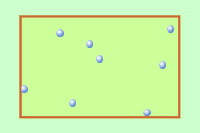This animation depicts thermal translational motions of molecules in a gas. In liquids and solids, there is vary little empty space between molecules, and they mostly just bump against and jostle one another.  [image]

You will notice that we have sneaked the the word "translational" into this definition of temperature. Translation refers to a change in location: in this case, molecules moving around in random directions. This is the major form of thermal energy under ordinary conditions, but molecules can also undergo other kinds of motion, namely rotations and internal vibrations. These latter two forms of thermal energy are not really "chaotic" and do not contribute to the temperature.

Energy is measured in joules, and temperature in degrees. This difference reflects the important distinction between energy and temperature:

• We can say that 100 g of hot water contains more energy (not heat!) than 100g of cold water. And because energy is an extensive quantity, we know that a 10-g portion of this hot water contains only ten percent as much energy as the entire 100-g amount.
• Temperature, by contrast, is not a measure of quantity; being an intensive  property, it is more of a "quality" that describes the "intensity" with which thermal energy manifests itself. So both the 100-g and 10-g portions of the hot water described above possess the same temperature.

### Temperature scales

Temperature is measured by observing its effect on some temperature-dependent variable such as the volume of a liquid or the electrical resistance of a solid. In order to express a temperature numerically, we need to define a scale which is marked off in uniform increments which we call degrees. The nature of this scale — its zero point and the magnitude of a degree, are completely arbitrary.

Although rough means of estimating and comparing temperatures have been around since AD 170, the first mercury thermometer and temperature scale were introduced in Holland in 1714 by Gabriel Daniel Fahrenheit.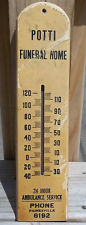Fahrenheit established three fixed points on his thermometer. Zero degrees was the temperature of an ice, water, and salt mixture, which was about the coldest temperature that could be reproduced in a laboratory of the time. When he omitted salt from the slurry, he reached his second fixed point when the water-ice combination stabilized at "the thirty-second degree." His third fixed point was "found as the ninety-sixth degree, and the spirit expands to this degree when the thermometer is held in the mouth or under the armpit of a living man in good health."

After Fahrenheit died in 1736, his thermometer was recalibrated using 212 degrees, the temperature at which water boils, as the upper fixed point. Normal human body temperature registered 98.6 rather than 96.

In 1743, the Swedish astronomer Anders Celsius devised the aptly-named centigrade scale that places exactly 100 degrees between the two reference points defined by the freezing- and boiling points of water.

For reasons best known to Celsius, he assigned 100 degrees to the freezing point of water and 0° to its boiling point, resulting in an inverted scale that nobody liked. After his death a year later, the scale was put the other way around. The revised centigrade scale was quickly adopted everywhere except in the English-speaking world, and became the metric unit of temperature. In 1948 it was officially renamed as the Celsius scale.

Why do we have so many temperature scales?

A short history of the thermometer and temperature scales

### Temperature comparisons and conversions

When we say that the temperature is so many degrees, we must specify the particular scale on which we are expressing that temperature. A temperature scale has two defining characteristics, both of which can be chosen arbitrarily:

• The temperature that corresponds to 0° on the scale;
• The magnitude of the unit increment of temperature — that is, the size of the degree.

In order to express a temperature given on one scale in terms of another, it is necessary to take both of these factors into account.

Converting between Celsius and Fahrenheit is easy if you bear in mind that between the so-called ice- and steam points of water there are 180 Fahrenheit degrees, but only 100 Celsius degrees, making the F° 100/180 = 5/9 the magnitude of the C°.

Because the ice point is at 32°F, the two scales are offset by this amount. If you remember this, there is no need to memorize a conversion formula; you can work it out whenever you need it.

Note the distinction between “°C” (a temperature) and “C°” (a temperature increment).

#### Absolute temperature scales

Near the end of the 19th Century when the physical significance of temperature began to be understood, the need was felt for a temperature scale whose zero really means zero — that is, the complete absence of thermal motion. This gave rise to the absolute temperature scale whose zero point is –273.15 °C, but which retains the same degree magnitude as the Celsius scale. This was eventually renamed after Lord Kelvin (William Thompson); thus the Celsius degree became the kelvin. It is now common to express an increment such as five C° as “five kelvins”

In 1859 the Scottish engineer and physicist William J. M. Rankine proposed an absolute temperature scale based on the Fahrenheit degree. Absolute zero (0° Ra) corresponds to –459.67°F. The Rankine scale has been used extensively by those same American and Brutish engineers who delight in expressing energies in units of BTUs and masses in pounds.

The importance of absolute temperature scales is that absolute temperatures can be entered directly in all the fundamental formulas of physics and chemistry in which temperature is a variable. Perhaps the most common example, known to all beginning students, is the ideal gas equation of state

PV = nRT.

4  Heat capacity

As a body loses or gains heat, its temperature changes in direct proportion to the amount of thermal energy q transferred:

q = C ΔT

The proportionality constant C is known as the heat capacity

C = q/ΔT

If ΔT is expressed in kelvins (degrees) and q in joules, the units of C are J K–1. In other words, the heat capacity tells us how many joules of energy it takes to change the temperature of a body by 1 C°. The greater the value of C, the the smaller will be the effect of a given energy change on the temperature.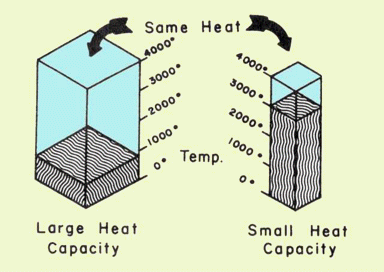It should be clear that C is an extensive property— that is, it depends on the quantity of matter. Everyone knows that a much larger amount of energy is required to bring about a 10-C° change in the temperature of 1 L of water compared to 10 mL of water. For this reason, it is customary to express C in terms of unit quantity, such as per gram, in which case it becomes the specific heat capacity, commonly referred to as the "specific heat" and has the units J K–1g–1.

[image]

↑ Thus if identical quantities of heat flow into two bodies having different heat capacities, the one having the smaller heat capacity will undergo the greater change in temperature. (You might find it helpful to think of heat capacity as a measure of a body's ability to resist a change of temperature when absorbing or losing heat.)

Note: you are expected to know the units of specific heat. The advantage of doing so is that you need not learn a "formula" for solving specific heat problems.